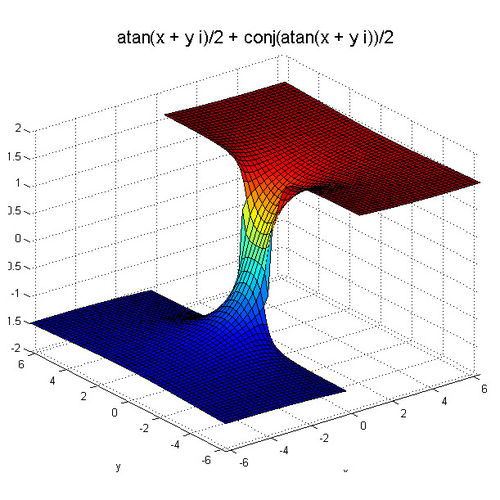# optimization software / mathematical calculationSymbolic Math Toolbox™

 Add to MyDirectIndustry favorites Compare this product Don’t compare this productoptimization software - Symbolic Math Toolbox™

## Characteristics

• Function:

optimization, mathematical calculation

## Description

Symbolic Math Toolbox™ provides functions for solving, plotting, and manipulating symbolic math equations. You can create, run, and share symbolic math code using the MATLAB® Live Editor. The toolbox provides libraries of functions in common mathematical areas such as calculus, linear algebra, algebraic and ordinary differential equations, equation simplification, and equation manipulation.Symbolic Math Toolbox lets you analytically perform differentiation, integration, simplification, transforms, and equation solving. Your computations can be performed either analytically or using variable-precision arithmetic, with the results displayed in mathematical typeset. You can share your symbolic work as live scripts with other MATLAB users or convert them to HTML or PDF for publication. You can generate MATLAB functions, Simulink® function blocks, and Simscape™ equations directly from symbolic expressions.